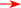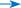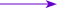Addition of vectors
Instruction: Drag two arrows representing two forces onto the graph paper. Press <Add> and observe how the two forces are added.

Add ResetΔt
Δv
Show acceleration
 Acceleration = slope of v–t graph =  ΔvΔt =  {{deltaV}}{{deltaT}} = {{acceleration}} m s−2